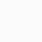# Electrostatic Potential

Chapter-2|Electrostatic Potential and Capacitance| NCERT 12th Physics:

Electrostatic Potential:

The electrostatic potential at any point in the region of electric field is equal to to the amount of work done in bringing a unit positive test charge (without acceleration) from infinity to that point.

Note:  The direction of flow of charge between two charged bodies placed in contact with each other is determined by electrostatic potential.

Electrostatic Potential Difference:

Let a point charge +q located at a point O. Let A and B be two points in its electric field. When a test charge q₀ is moved from A to B, a work has to be done in moveing against the repulsive force exerted by the charge +q. We then calculate the potential difference between point A and B by the equation.

So, the potential difference between two points in an electric field may be defined as the amount of work done in moving an unit positive charge from one point to the other against the electrostatic forces.

Hence, the potential difference between two points in an electric field it is said to be one volt if one joule of work has to be done in moving a positive charge of one coulomb from one point to the other against the electrostatic forces.

## 1 thought on “Electrostatic Potential”

1.Unknown says:

Nic e